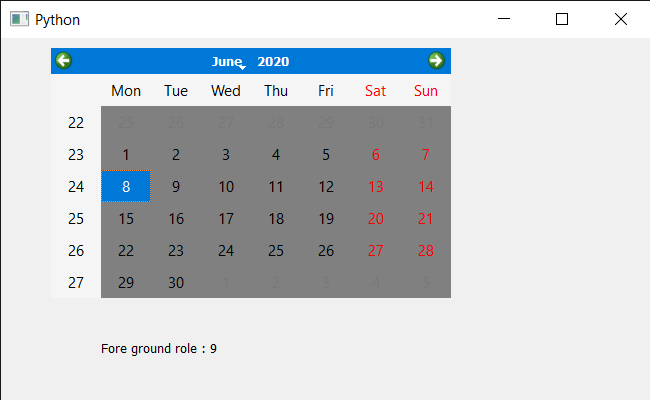GFG App
Open AppBrowser
Continue

# PyQt5 QCalendarWidget – Getting Foreground Role

In this article, we will see how we can get the foreground role of the QCalendarWidget. There are basically two types of roles in the calendar one is foreground and the other one is background. The foreground role defines the color from the calendar’s palette that is used to draw the foreground. If no explicit foreground role is set, the foreground returns a role that contrasts with the background role. We can set the foreground role with the help of setForegroundRole method.

In order to do this we will use foregroundRole method with the QCalendarWidget object.
Syntax : calendar.foregroundRole()
Argument : It takes no argument
Return : It return QPalette.ColorRole object but when printed it shows the value associated with the role

Below is the implementation

## Python3

 `# importing libraries ` `from` `PyQt5.QtWidgets ``import` `*` `from` `PyQt5 ``import` `QtCore, QtGui ` `from` `PyQt5.QtGui ``import` `*` `from` `PyQt5.QtCore ``import` `*` `import` `sys `     `class` `Window(QMainWindow): `   `    ``def` `__init__(``self``): ` `        ``super``().__init__() `   `        ``# setting title ` `        ``self``.setWindowTitle(``"Python "``) `   `        ``# setting geometry ` `        ``self``.setGeometry(``100``, ``100``, ``650``, ``400``) `   `        ``# calling method ` `        ``self``.UiComponents() `   `        ``# showing all the widgets ` `        ``self``.show() `   `    ``# method for components ` `    ``def` `UiComponents(``self``): ` `        ``# creating a QCalendarWidget object ` `        ``self``.calendar ``=` `QCalendarWidget(``self``) `   `        ``# setting geometry to the calendar ` `        ``self``.calendar.setGeometry(``50``, ``10``, ``400``, ``250``) `   `        ``# setting cursor ` `        ``self``.calendar.setCursor(Qt.PointingHandCursor) `   `        ``# setting foreground role ` `        ``self``.calendar.setForegroundRole(QPalette.Base) `   `        ``# getting palette and setting color to the background to it ` `        ``p ``=` `self``.calendar.palette() ` `        ``p.setColor(``self``.calendar.foregroundRole(), Qt.darkGray) ` `        ``self``.calendar.setPalette(p) `   `        ``# creating label to show the properties ` `        ``self``.label ``=` `QLabel(``self``) `   `        ``# setting geometry to the label ` `        ``self``.label.setGeometry(``100``, ``280``, ``250``, ``60``) `   `        ``# making label multi line ` `        ``self``.label.setWordWrap(``True``) `   `        ``# getting foreground role ` `        ``value ``=` `self``.calendar.foregroundRole() `   `        ``# setting text to the label ` `        ``self``.label.setText(``"Fore ground role : "` `+` `str``(value)) `     `# create pyqt5 app ` `App ``=` `QApplication(sys.argv) `   `# create the instance of our Window ` `window ``=` `Window() `   `# start the app ` `sys.exit(App.``exec``()) `

Output :My Personal Notes arrow_drop_up